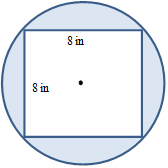Chapter 8.4, Problem 23EElementary Geometry For College St...

7th Edition
Alexander + 2 others
ISBN: 9781337614085

Solutions

Chapter
SectionElementary Geometry For College St...

7th Edition
Alexander + 2 others
ISBN: 9781337614085
Textbook Problem

In exercises 23-26, find the exact areas of shaded region.Square inscribed in a circle

To determine

To Find:

The areas of the shaded region.

Explanation

To find the area of the shaded region of the circle, find the area of the circle and square and then subtract the area of the square from the area of the circle.

It is given that the side of the square is 8 in.

Therefore, the area of the square is A=s2.

Therefore, A=88=64.

The area of the square is 64 in2.

Next, to find the area of the circle, we need radius of the circle.

The radius can be obtained from the diagonal of the square.

The diagonal of the square is 2s.

Therefore, the diagonal of the square is 82.

The diagonal of the square is equal to the diameter of the circle.

Therefore, the diameter of the circle is 82.

The radius of the circle is r=d2=822=42

Still sussing out bartleby?

Check out a sample textbook solution.

See a sample solution

The Solution to Your Study Problems

Bartleby provides explanations to thousands of textbook problems written by our experts, many with advanced degrees!

Get Started

Differentiate. y=cosx1sinx

Single Variable Calculus: Early Transcendentals, Volume I

In Problems 29-32, use algebra to rewrite the integrands; then integrate and simplify. 32.

Mathematical Applications for the Management, Life, and Social Sciences

Sometimes, Always, or Never: The antiderivative of an elementary function is elementary.

Study Guide for Stewart's Single Variable Calculus: Early Transcendentals, 8th